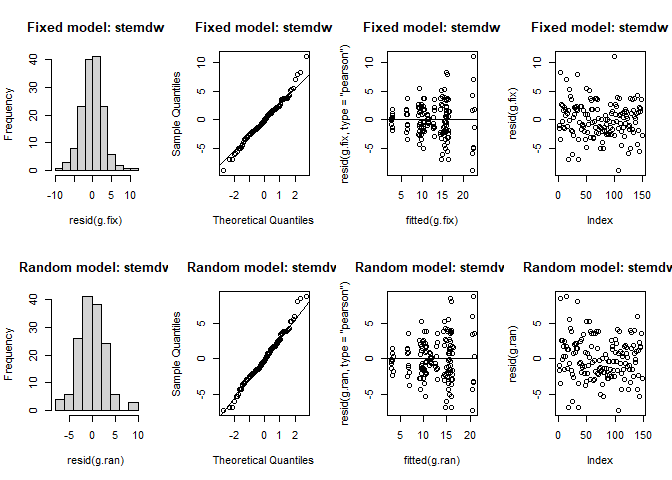# Broad-sense heritability ($$H^2$$)

Broad-sense heritability ($$H^2$$) is defined as the proportion of phenotypic variance that is attributable to an overall genetic variance for the genotype . There are usually additional interpretations associated with $$H^2$$: (i) It is equivalent to the coefficient of determination of a linear regression of the unobservable genotypic value on the observed phenotype; (ii) It is also the squared correlation between predicted phenotypic value and genotypic value; and (iii) It represents the proportion of the selection differential ($$S$$) that can be realized as the response to selection ($$R$$) .

There are two main reasons why heritability on an entry-mean basis is of interest in plant breeding :

1. It is plugged into the breeder’s Equation to predict the response to selection.
2. It is a descriptive measure used to assess the usefulness and precision of results from cultivar evaluation trials.

## Breeder´s equation

$\Delta G=H^2S$ Where:

• $$\Delta G$$ is the genetic gain
• $$S$$ is the mean phenotypic value of the selected genotypes, expressed as a deviation from the population mean.

# Usual Problems

In practice, most trials are conducted in a multienvironment trial (MET) presente unbalanced data as not all cultivars are tested at each environment or simply when plot data is lost or when the number of replicates at each location varies between genotypes . However, the standard method for estimating heritability implicitly assumes balanced data, independent genotype effects, and homogeneous variances.

# How calculate the Heritability?

According Schmidt et al. (2019a), the variance components could be calculated in two ways:

## 1) Two stages approach

For the two stage approach, in the first stage each experiment is analyzed individually according their experiment design (Lattice, CRBD, etc) . And for the second stage environments are denotes a year-by-location interaction. This approach assumes a single variance for genotype-by-environment interactions (GxE), even when multiple locations were tested across multiple years .

### Model

$y_{ikt}=\mu\ +\ G_i+E_t+GxE_{it}+\varepsilon_{ikt}$

### Phenotypic variance

$\sigma_p^2=\sigma_g^2+\frac{\sigma_{g\cdot e}^2}{n_e}+\frac{\sigma_{\varepsilon}^2}{n_e\cdot n_r}$

## 2) One stage approach

For the one stage approach only one model is used for the MET analysis. The environmental effects are included via separate year, and location main interaction effects.

$y_{ikt}=\mu+G_i+Y_m+E_q+YxE_{mq}+GxY_{im}+GxE_{iq}+GxYxE_{imq}+\varepsilon_{ikmq}$

### Phenotypic variance

$\sigma_p^2=\sigma_g^2+\frac{\sigma_{g\cdot e}^2}{n_e}+\frac{\sigma_{g\cdot y}^2}{n_y}+\frac{\sigma_{g\cdot y\cdot e}^2}{n_y\cdot n_e}+\ \frac{\sigma_{\epsilon}^2}{n_e\cdot n_y\cdot n_r}$

# Differentes heritability calculations

Table 1: Differentes heritability calculation
Standart Cullis Piepho
$$H^2=\frac{\sigma_g^2}{\sigma_p^2}=\frac{\Delta G}{S}$$ $$H_{Cullis}^2=1-\frac{\overline{V}_{\Delta..}^{^{BLUP}}}{2\cdot\sigma_g^2}$$ $$H_{Piepho}^2=\frac{\sigma_g^2}{\sigma_g^2+\frac{\overline{V}_{\Delta..}^{BLUE}}{2}}$$

# Heritability function in the package

For calculate the standard heritability in MET experiments the number of location and replication should be include manually in the function H2cal(). In the case of difference number of replication in each experiments, take the maximum value (often done in practice) .

For remove the outliers the function implemented is the Method 4 used for Bernal-Vasquez et al. (2016): Bonferroni-Holm using re-scaled MAD for standardizing residuals (BH-MADR).

library(inti)

## H2cal function

 dt <- potato
hr <- H2cal(data = dt
, trait = "stemdw"
, gen.name = "geno"
, rep.n = 5
, fixed.model = "0 + (1|bloque) + geno"
, random.model = "1 + (1|bloque) + (1|geno)"
, emmeans = TRUE
, plot_diag = TRUE
, outliers.rm = TRUE
)hr$model %>% summary() ## Linear mixed model fit by REML ['lmerMod'] ## Formula: stemdw ~ 1 + (1 | bloque) + (1 | geno) ## Data: dt.rm ## Weights: weights ## ## REML criterion at convergence: 796.1 ## ## Scaled residuals: ## Min 1Q Median 3Q Max ## -2.38440 -0.64247 -0.08589 0.57452 2.84508 ## ## Random effects: ## Groups Name Variance Std.Dev. ## geno (Intercept) 19.960 4.4677 ## bloque (Intercept) 0.110 0.3316 ## Residual 9.411 3.0677 ## Number of obs: 148, groups: geno, 15; bloque, 5 ## ## Fixed effects: ## Estimate Std. Error t value ## (Intercept) 12.51 1.19 10.51 ## Variance components hr$tabsmr %>% kable(caption = "Variance component table")
Table 2: Variance component table
trait rep geno env year mean std min max V.g V.e V.p repeatability H2.s H2.p H2.c
stemdw 5 15 1 1 12.59867 4.749994 2.818 22.302 19.96002 9.410932 21.84221 0.913828 0.913828 0.9502395 0.9533473

Table 4: BLUPs
geno stemdw
G01 15.587018
G02 10.228658
G03 9.821839
G04 15.057007
G05 12.843686
G06 20.631268
G07 3.254483
G08 10.517060
G09 15.525899
G10 9.389236
G11 6.699074
G12 15.948953
G13 14.533681
G14 16.126578
G15 11.515963

## Outliers

hr$outliers$fixed %>% kable(caption = "Outliers fixed model")
Table 5: Outliers fixed model
68 IV G05 80.65 60.36709 18.84505 0 68 0 0 OUTLIER
hr$outliers$random %>% kable(caption = "Outliers random model")
Table 6: Outliers random model
68 IV G05 80.65 61.39925 18.886677 0.0000000 68 0.0000000000 0.0000000 OUTLIER
100 IV G06 33.52 12.02340 3.698449 0.0002169 100 0.0002169207 0.0323212 OUTLIER

# Comparison: H2cal and asreml

https://inkaverse.com/articles/extra/stagewise.html

Bernal-Vasquez, A.-M., H.-F. Utz, and H.-P. Piepho. 2016. Outlier detection methods for generalized lattices: A case study on the transition from ANOVA to REML. Theoretical and Applied Genetics 129(4): 787–804. doi: 10.1007/s00122-016-2666-6.
Bolker, B. 2021. Mean variance of a difference of BLUEs or BLUPs in lme4. Stack Overflow. https://stackoverflow.com/questions/38697477/mean-variance-of-a-difference-of-blues-or-blups-in-lme4 (accessed 21 May 2021).
Buntaran, H., H.-P. Piepho, P. Schmidt, J. Rydén, M. Halling, et al. 2020. Cross-validation of stagewise mixed-model analysis of Swedish variety trials with winter wheat and spring barley. Crop Science 60(5): 2221–2240. doi: 10.1002/csc2.20177.
Falconer, D.S., and T.F. Mackay. 2005. Introduction to quantitative genetics (Pearson Prentice Hall, editor). Fourth.
Schmidt, P., J. Hartung, J. Bennewitz, and H.-P. Piepho. 2019a. Heritability in Plant Breeding on a Genotype-Difference Basis. Genetics 212(4): 991–1008. doi: 10.1534/genetics.119.302134.
Schmidt, P., J. Hartung, J. Rath, and H.-P. Piepho. 2019b. Estimating Broad-Sense Heritability with Unbalanced Data from Agricultural Cultivar Trials. Crop Science 59(2): 525–536. doi: 10.2135/cropsci2018.06.0376.
Zystro, J., M. Colley, and J. Dawson. 2018. Alternative Experimental Designs for Plant Breeding. Plant Breeding Reviews. John Wiley & Sons, Ltd. p. 87–117